# Team:TAS Taipei/Modeling

Modeling - TAS Taipei iGEM Wiki

# Modeling

Granzyme B (GzmB) activity is elevated during inflammation, which can lead to excess cleavage of extracellular matrix (ECM) proteins in human tissue. Aside from causing damage to healthy tissues, this also exacerbates chronic inflammatory conditions, which keeps producing more GzmB and creates a vicious cycle. Our project aims to selectively inhibit GzmB in the ECM in order to prevent damage during chronic inflammation. Since we do not have the means of working with GzmB and directly testing its interactions in human test subjects, we predicted these interactions using mathematical models and data from the literature.

The Hill equation is used to model the interaction between a ligand and its binding partner, a macromolecule/enzyme – in our case, how well a GzmB inhibitor binds to GzmB. It is a function of free ligand concentration, and it returns the fraction of macromolecules that are bound. Two constants are involved in this equation: the dissociation constant and the Hill coefficient (Weiss, 1997).

The Hill coefficient describes cooperativity of the binding. Positive cooperativity (n>1) means that a bound enzyme has higher affinity for other ligands; negative cooperativity (n<1) means the converse is true; and non-cooperative binding (n=0) means that whether or not the enzyme is bound has no bearing on further binding (Weiss, 1997).

The Hill equation is shown below: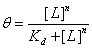, where [L] is concentration of the ligand (in our case, the inhibitor), Kd is the dissociation constant, and n is the Hill coefficient.

## Mouse Inhibitor Serpina3n vs GzmB Activity

Our first dataset (Table 1), which measures Serpina3n concentration vs relative GzmB activity, came from research by Ang et al. at the University of British Columbia (2011). Serpina3n is a known mouse GzmB inhibitor, and the paper documented the effects of Serpina3n on GzmB activity. Given its specificity in mice, this inhibitor was not chosen as part of the final construct, but data from this paper was used because the relevant constants can be analyzed and compared to other inhibitor substitutes.

 [Sa3n] (nM) 0.4 1.4 2.8 3.8 5 7.8 10 16 21 40 80 Relative GzmB Activity (%) 118 110 115 102 78 76 65 56 39 15 4

Several adjustments were made to the equation and dataset so that we could have a meaningful model. First, GzmB activity was shown as percentages ranging from 10% to 120% in the paper. This is likely because the control for the experiment was set at some point where the Serpina3n expression is not 0. Thus, we scaled down the data points by a factor of 1/120. This scaling is necessary since the Hill equation returns a fraction; the output is limited to between 0 and 1.

More importantly, since the data was presented as the concentration of enzymes that remain free after binding, we needed to modify the Hill equation in order to model the fraction of unbound GzmB. Since bound + unbound = 100%, and the Hill equation models the bound component, it can be said that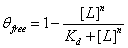, provided that the dataset is properly scaled to a range between 100%-0%.

The FindFit function of Mathematica was used to find the unknown constants Kd and n for the modified Hill equation above. Our model returned a Kd of ≈ 28.130 and n ≈ 1.3540. The final equation is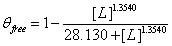, where θfree is GzmB activity and [L] stands for concentration of Serpina3n (Figure 1).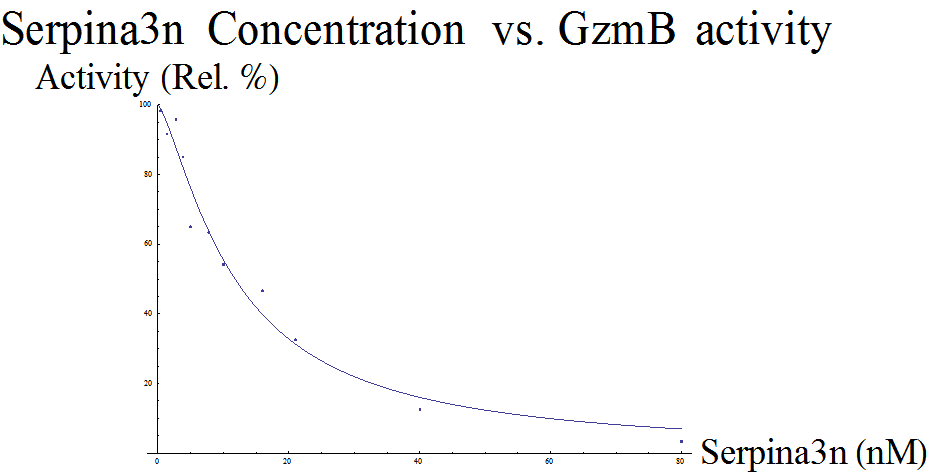Figure 1. Model: Serpina3n Inhibition of GzmB. Using data from the Ang et al. paper, we developed an equation that models relative GzmB Activity as a function of Serpina3n concentration

## Human GzmB Inhibitor ACT3m

Given our model of Serpina3n, we also wanted to create a model for the protein used in our device, ACT3m. The dataset obtained from the ACT3m paper (Marcet-Palacios et al., 2014) is the result of a colorimetric assay. Data was presented as absorbance values (A405), which correspond to the concentration of free GzmB, at different inhibitor concentrations. The paper used this to prove that their novel ACT3m inhibitor was the strongest out of their entire pool of possible candidates: treatment with ACT3m resulted in the lowest A405 values, which suggests the strongest inhibition of GzmB.

To model this set of data, similar adjustments had to be made. First, the dataset was scaled so the numbers ranged between 0 and 1 (in this case, increased by a factor of 2). The same modified Hill equation was used from the Serpina3n analysis. Our model returned a Kd of ≈ 13.336 and n ≈ 0.605, so the equation looks like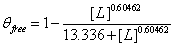(Figure 2).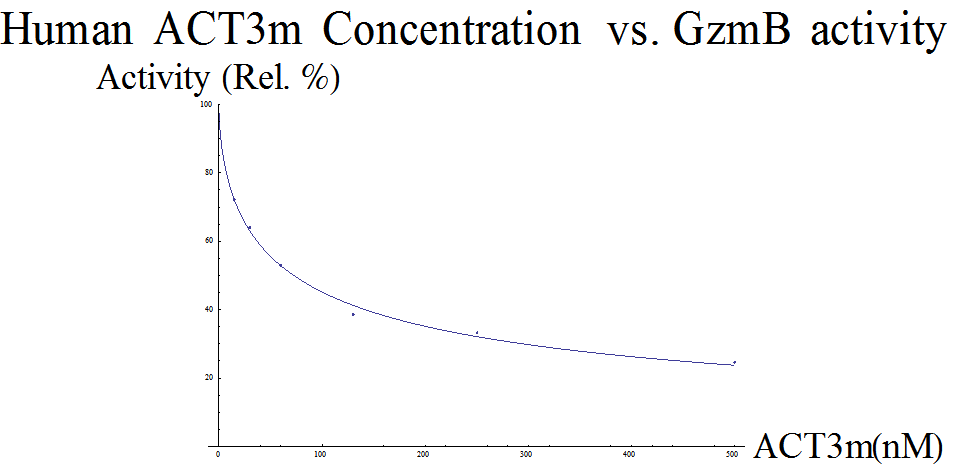Figure 2. Model: ACT3m Inhibition of GzmB. Using data from the Marcet-Palacios et al. paper, we developed an equation that models relative GzmB activity as a function of ACT3m concentration.concentration.

## Analysis

Table 2. Values Returned from Model: the dissociation, association and Hill constants were calculated using Mathematica.
Kd (nM) Ka (nM^-1) n
Sa3n 28.130 0.035549 1.3540
ACT3m 13.336 0.074985 0.60462

To interpret the above data, we first compare the constants of dissociation, Kd. In this context, Kd represents [Gzmbfree][Inhibitor]/[Gzmbinhib-Inhibitor] at equilibrium (Bisswanger, 2008); therefore, a lower Kd would represent better inhibition: more bound GzmB compared to free GzmB.

We can also analyze the Ka, the association constant. Ka is the inverse of Kd, and can be found as [Gzmbinhib-Inhibitor]/ [Gzmbfree][Inhibitor]. This represents the relative amount of bound molecules, so that a higher Ka represents stronger binding affinity. Compared to the mouse inhibitor Serpina3n, human ACT3m has a lower Kd and a higher Ka, which suggests stronger inhibition. This conclusion is in agreement with Marcet-Palacios et al., where this novel inhibitor was also compared to mouse Serpina3n (2014).

The Hill constant may also be considered to analyze the nature of the inhibitor-GzmB complex. With n>1, Serpina3n is likely to bind to multiple sites on GzmB (Weiss et al., 1997). ACT3m, in contrast, has a n<1, meaning that it likely binds competitively to selective and perhaps singular sites. This information is interesting; taking the association constants into account, this means that despite less opportunity to bind, ACT3m still acts as a better inhibitor than Serpina3n.

## Inhibitor Concentration Calculator

The following equations were developed with a question in mind:

### How much inhibitor is needed to bring GzmB levels back to normal?

Since there are many diseases that cause inflammation, and thus increased levels of GzmB, we created a calculator to determine the amount of treatment needed for any GzmB-related diseases. The model can show the relative percent decrease of GzmB as the inhibitor concentration increases. A patient could obtain information regarding their condition and calculate the amount of treatment needed.

Given that, reversing the parameters yields the equivalent function of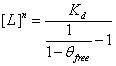, or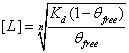. This equation calculates the inhibitor concentration that corresponds to a certain relative level of GzmB.

Rheumatoid arthritis (RA) is one of the main chronic inflammatory diseases made worse by elevated GzmB activity. We will use this as an example to show how the calculator works. GzmB concentrations in synovial fluids (joint fluids) of arthritis patients were determined from Tak et al., 2009 (summarized in Table 3). There is a significant GzmB concentration difference between the control group and RA patients. The equation that returns a concentration of inhibitor can be used here, as it takes in relative GzmB levels and returns the amount of inhibitor needed.

Table 3: GzmB Concentration in Synovial Fluids. GzmB concentration in patients suffering from rheumatoid arthritis obtained from Tak et al., 2009.
Rheumatoid arthritis (pg/mL) Control (pg/mL)
Mean +/- s.d. 3306 +/- 10311 34 +/- 32
Median 251 29

If a patient has a GzmB level that is X times the amount of control (around 34 pg/mL), then proportionally, X/100% = 100%/Y, where Y is the factor we want to decrease the patient’s level by (relative to his original level). Therefore,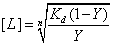, and the amount of inhibitor needed [L] can be calculated. For example, if an RA patient has a GzmB concentration of 3400 pg/mL, then the patient has about 100x the normal GzmB level. Therefore, the Y factor would be 1%, and [L] can be calculated to be around 130 uM.

It should be noted, however, that there is a large standard deviation for GzmB levels in RA patients, which means there are significant variations: if treatments are to be given, it would be better to conduct treatments on a case-by-case basis.

#### CALCULATOR

Patient's GzmB level is % of original
Treatment ([L]) :        nM of ACT

## Bandage Flux Model

Now that we know how to calculate the amount of treatment necessary, we can estimate how we apply our prototype to deliver the appropriate amount of treatment.

Fick’s first law dictates that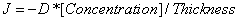, where J is the diffusion rate (flux) across the membrane (Lonsdale et al.). This models negative flux entering a closed surface. For our model, we want to model the flux that leaves the closed surface, which is our bandage. Thus, we use the model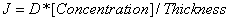, where J is a positive flux.

[Concentration] is the concentration of molecules inside the bandage, in our case the GzmB inhibitor. The thickness of the membrane is inversely related to J. D is the diffusivity, or the diffusion constant, of a certain configuration – a greater D generally represents a greater rate of diffusion. In our model, the diffusivity of our cellulose ester membrane was found to be 5.7x10^-6 cm^2/sec. Therefore, our diffusion model is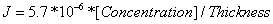.

When graphed, the 3-dimensional equation looks like Figure 3 below: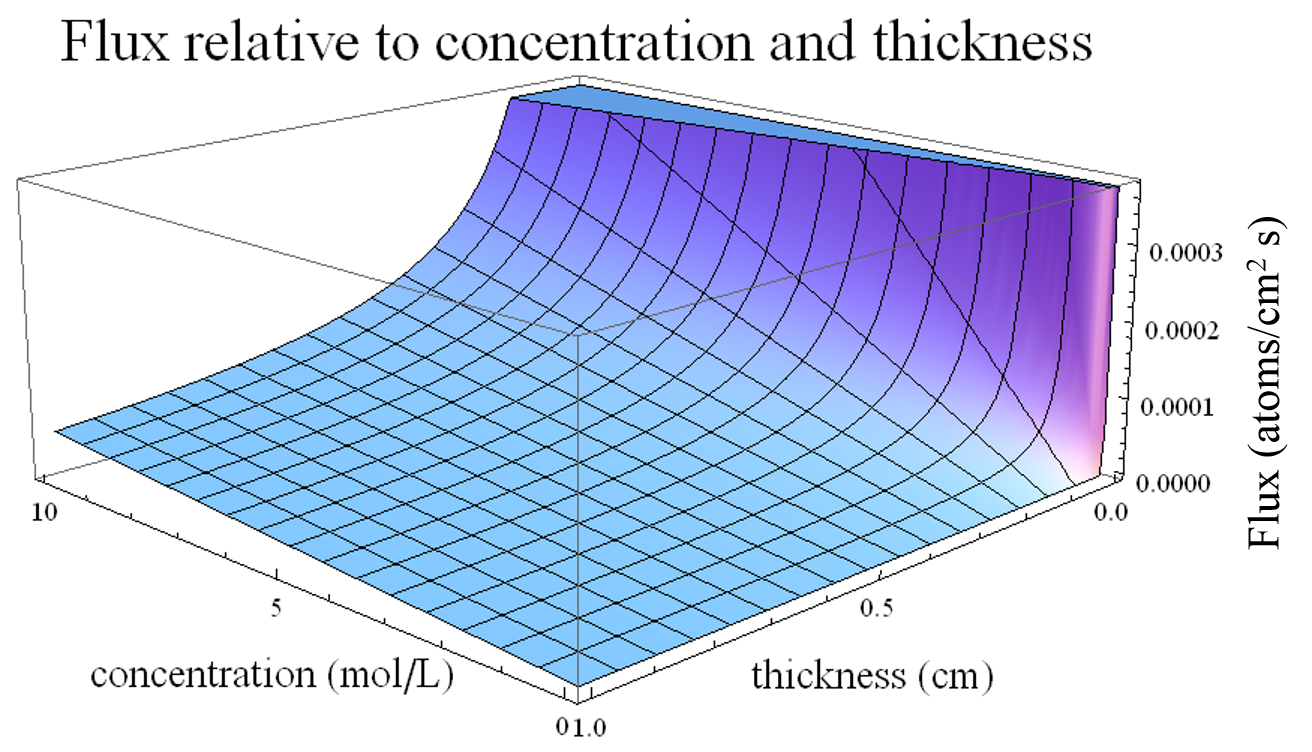Figure 3. Flux relative to concentration and membrane thickness. Fick's first law was used to predict the rate of flow across the membrane.

As demonstrated by the graph, thickness is inversely related to the diffusion rate; on the other hand, concentration is directly related to the diffusion rate. As part of future work, we can try to combine this model with the calculator to find proper treatment concentration, perhaps mathematically integrating the flux to determine an effective concentration that the bandage delivers. However, this would likely require experimental data to verify the accuracy of our current models.

### Citations

Weiss, J. (1997). The Hill equation revisited: Uses and misuses. Faseb J, 11(11), 835-841. Retrieved September 5, 2015, from Pubmed.

Ang, L., Boivin, W., Williams, S., Zhao, H., Abraham, T., Carmine-Simmen, K., Granville, D. (2011). Serpina3n attenuates granzyme B-mediated decorin cleavage and rupture in a murine model of aortic aneurysm. Cell Death Dis Cell Death and Disease.

Marcet-Palacios, M., Ewen, C., Pittman, E., Duggan, B., Carmine-Simmen, K., Fahlman, R., & Bleackley, R. (2014). Design and characterization of a novel human Granzyme B inhibitor. Protein Engineering Design and Selection, 9-17.

Bisswanger, H. (2008). Enzyme kinetics: Principles and methods (2nd rev. and updated ed.). Weinheim: Wiley-VCH.

Tak PP, Spaeny-Dekking L, Kraan MC, Breedveld FC, Froelich CJ, Hack CE. The levels of soluble granzyme A and B are elevated in plasma and synovial fluid of patients with rheumatoid arthritis (RA). Clinical and Experimental Immunology. 1999;116(2):366-370. doi:10.1046/j.1365-2249.1999.00881.x.

Vaughan, M. (1959). Cellulose Acetate Membranes suitable for Osmotic Measurements. Nature, 43-44.